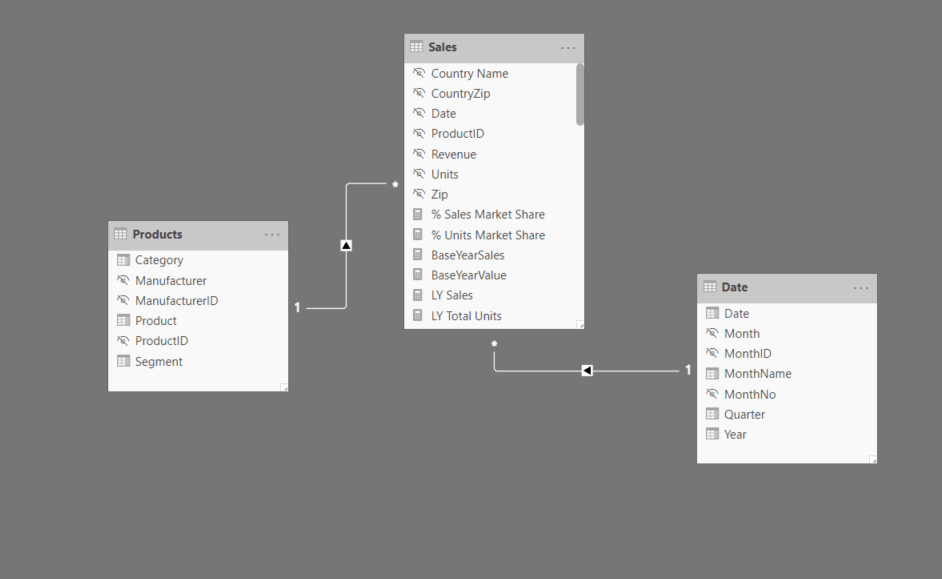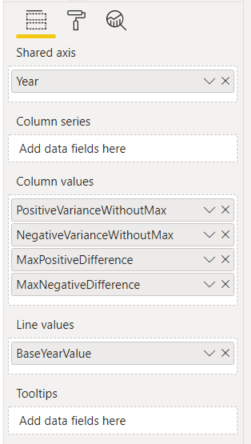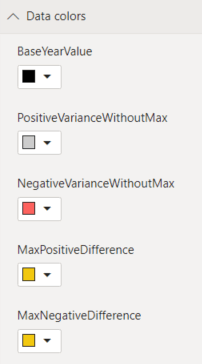#### +61 4 3836 7017

This is the fifth post in the Strategic colouring in Power BI series. Links to all the posts can be found here

https://www.capstoneanalytics.com.au/master-post-for-strategic-colouring-articles/

In the fourth post, we discussed how to isolate and visualise YTD data

In this post we will discuss how to visualise differences in sales for various categories in a field (Segment) across all the years in a model from a base year.

Our model has one fact table Sales and two dimension tables Date and ProductDefine Total Sales as

Total Sales =
SUM ( Sales[Revenue] )

Next we will create a copy of the Date table and call it Date 2 and define these three measures

SelectedBaseYear =
SELECTEDVALUE ( ‘Date 2′[Year] )

BaseYearValue =
IF ( [SelectedBaseYear] = SELECTEDVALUE ( ‘Date'[Year] )0BLANK () )

BaseYearSales =
CALCULATE ( [Total Sales], TREATAS ( VALUES ( ‘Date 2′[Year] ), ‘Date'[Year] ) )

SelectedBaseYear gives the Year when selected from a slicer. BaseYearValue will be used to highlight the SelectedBaseYear in the chart. BaseYearSales gives the Total Sales for the SelectedBaseYear

Now we define the variance measures

VarianceFromBaseYearNegative =
VAR diff = [Total Sales] – [BaseYearSales]
RETURN
IF ( diff < 0diff0 )

VarianceFromBaseYearPositive =
VAR diff = [Total Sales] – [BaseYearSales]
RETURN
IF ( diff > 0diff0 )

We also need to calculate the Maximum and Minimum differences so that we can highlight them in the chart

MaxPositiveDifference =
VAR baseyearsales = [BaseYearSales]
VAR result =
CALCULATE (
MAXX (
SUMMARIZE ( Sales, ‘Date'[Year], “Variance”, [Total Sales] – baseyearsales ),
[Variance]
),
ALL ( ‘Date'[Year] )
)
RETURN
IF ( result = [VarianceFromBaseYearPositive], result0 )

MaxNegativeDifference =
VAR baseyearsales = [BaseYearSales]
VAR result =
CALCULATE (
MINX (
SUMMARIZE ( Sales, ‘Date'[Year], “Variance”, [Total Sales] – baseyearsales ),
[Variance]
),
ALL ( ‘Date'[Year] )
)
RETURN
IF ( result = [VarianceFromBaseYearNegative], result0 )

Now we need to calculate two more measures so that we dont double up on the numbers

PositiveVarianceWithoutMax =
IF (
[VarianceFromBaseYearPositive] = [MaxPositiveDifference],
0,
[VarianceFromBaseYearPositive]
)

NegativeVarianceWithoutMax =
IF (
[VarianceFromBaseYearNegative] = [MaxNegativeDifference],
0,
[VarianceFromBaseYearNegative]
)

Drag a Line and Stacked Column chart into a blank canvas and drag the following fields (Year is from Date table)The colours are formatted to something similarNow drag Year from Date 2 table and drag it into a slicer and make it single select

Also drag Segment from Product table and drag it into a slicer and make it single select

Now you can select the Year which will serve as the Base Year and select different categories in the Segment field to look at the variances.

Link to interactive report here

Share this